Computer Science

# If statements

Imagine you have Bit starting in this world:You want to change every red square into a blue square: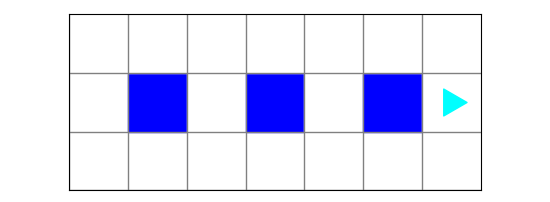You might think you could do this:

``````while bit.front_clear():
bit.move()
bit.paint('blue')
bit.move()``````

This works, but what if you want your code to work no matter how many red dots there are? For example, you could have a world like this: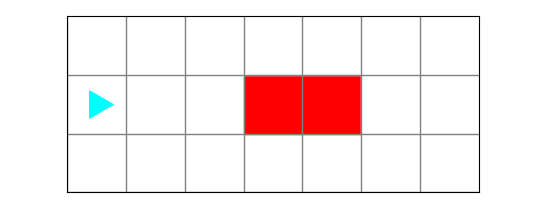or this: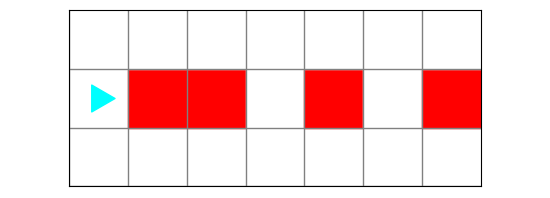## if statement

This is where we need to use an `if` statement. We want Bit to move from left to right and if it encounters a red square, change it to blue:

``````if bit.is_red():
bit.paint('blue')``````

These are the parts of an `if` statement: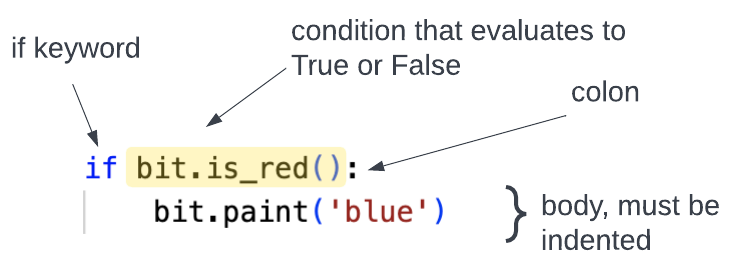• it must start with the `if` keyword
• this is followed by a condition that evaluates to True or False
• the first line ends with a colon `:`
• the body of the `if` statement is indented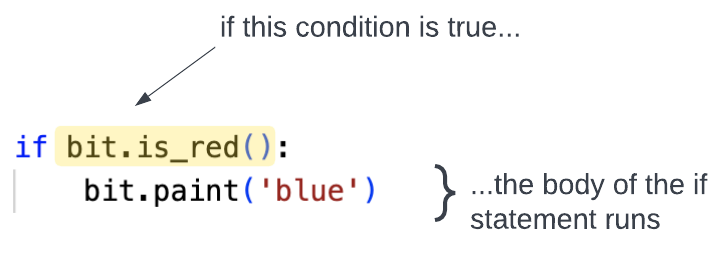Python checks the condition in the `if` statement. If it is true, then it runs the lines of code in the body of the loop. If it is not true, then it does not run the code in the body of the loop — it skips over it.

To see this in action, download the zip file listed above and put it in your `bit` folder. Look at the file called `red-dots.py`:

``````from byubit import Bit

def change_square(bit):
if bit.is_red():
bit.paint('blue')

@Bit.worlds('red-dots', 'another-red-dots', 'yet-another-red-dots')
def go(bit):
while bit.front_clear():
bit.move()
change_square(bit)

if __name__ == '__main__':
go(Bit.new_bit)
``````

This code loads all three Bit worlds shown above. The function called `go()` moves forward as long as the front is clear. The function called `change_square()` changes a square to blue if it is red.

Click the tabs for the different worlds, and you will see that this code works for all three worlds.

## Event stream pattern

This code follows the `event stream` pattern. The idea behind this pattern is that we use the `while` statement to move through the world and then the `if` statement to modify the world, depending on some condition.

In the code above:

• `go()` — moves Bit forward
• `change_squares()` — modifies the world based on conditions

## Using else with if

Let’s take a look at another Bit world:This world has some red squares and some green squares. The job for Bit this time is to turn red squares to blue and to turn any blank squares into green squares: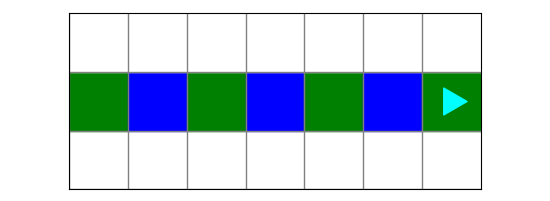We can do this with an `if-else` statement:

``````if bit.is_red():
bit.paint('blue')
else:
bit.paint('green')``````

This tells Bit to check if a square is red. If it is red, Bit paints it blue. Otherwise, Bit paints the square green.

These are the parts of an `if-else` statement: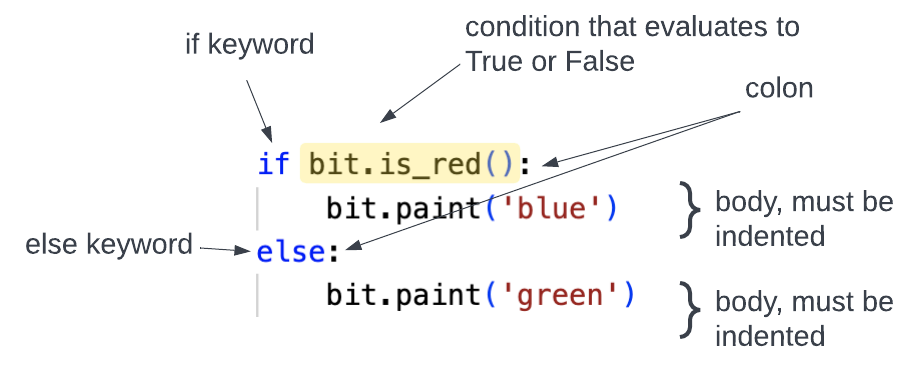• `if`, a condition, and a colon
• a block for the `if`
• `else`, a colon
• a block for the `else`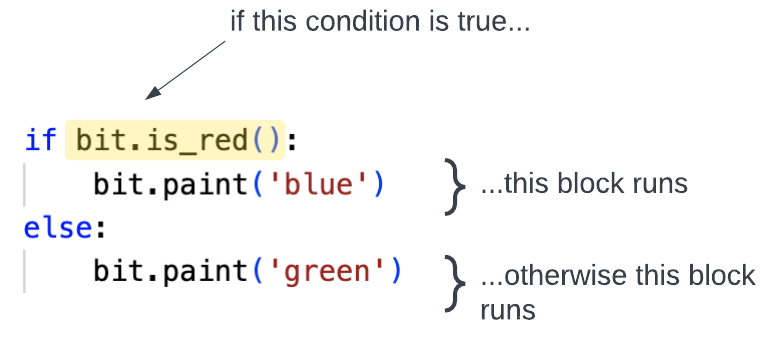To see this in action,look at the file called `more-red-dots.py`. We again use the `event stream` pattern:

``````from byubit import Bit

def change_squares(bit):
if bit.is_red():
bit.paint('blue')
else:
bit.paint('green')

@Bit.worlds('more-red-dots')
def go(bit):
while bit.front_clear():
bit.move()
change_squares(bit)

if __name__ == '__main__':
go(Bit.new_bit)
``````

Step through the code with `First` and `Next` to see how this works.

## Using elif with if

Take a look at this world:Bit needs to follow these rules:

• while Bit is not blocked, move forward
• if the current square is red, turn left
• if the current square is green, turn right
• otherwise, paint the current square blueTo have Bit follow these rules, we are going to use `if`, `elif`, `else`:

``````    if bit.is_red():
bit.left()
elif bit.is_green():
bit.right()
else:
bit.paint('blue')``````

This is how you can use these together:• `if`, a condition, and a colon
• a block for the `if`
• `elif`, a condition, and a colon
• a block for the `elif`
• `else`, a colon
• a block for the `else`

Python will check each of the conditions in order: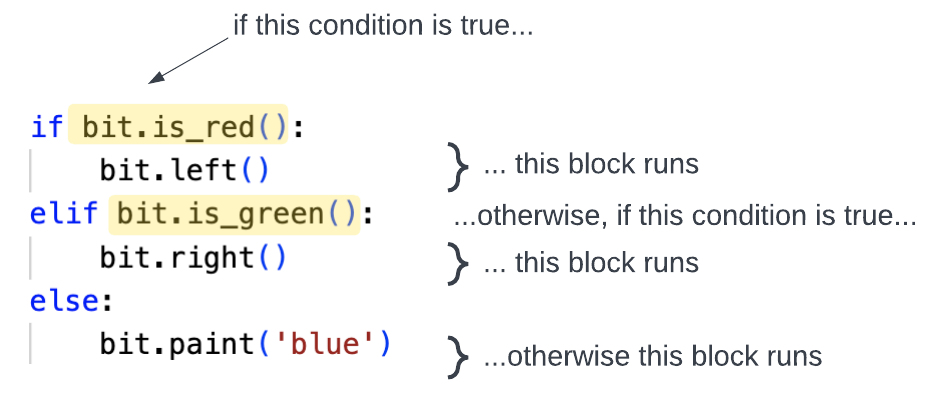You can add as many `elif` conditions as you want. There can be only one `if` and one `else` block.

To see this code in action, open the `turns.py` file:

``````from byubit import Bit

def respond_to_square(bit):
if bit.is_red():
bit.left()
elif bit.is_green():
bit.right()
else:
bit.paint('blue')

@Bit.worlds('turns')
@Bit.pictures()
def go(bit):
bit.paint('blue')
while bit.front_clear():
bit.move()
respond_to_square(bit)

if __name__ == '__main__':
go(Bit.new_bit)
``````

Run the code using the `First` and `Next` buttons to watch how it works, step by step.